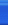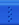Focus on bigdata and cloud.人们对复杂的软件系统常有一种处理手法，即增加一层间接层，从而对系统获得一种更为灵活、在面向对象系统中，有些对象由于某种原因（比如对象创建的开销很大，或者某些操作需要安全控制，或者需要进程外的访问等），直接访问会给使用者、或者系统结构带来很多麻烦。

如何在不失去透明操作对象的同时来管理/控制这些对象特有的复杂性？增加一层间接层是软件开发中常见的解决方式。

为其他对象提供一种代理以控制对这个对象的访问。  -------《设计模式》GOF1 public class Math
2 {
3     public double Add(double x,double y)
4     {
5         return x + y;
6     }
7
8     public double Sub(double x,double y)
9     {
10         return x - y;
11     }
12
13     public double Mul(double x,double y)
14     {
15         return x * y;
16     }
17
18     public double Dev(double x,double y)
19     {
20         return x / y;
21     }
22 }1 public class App
2 {
3     public static void Main()
4     {
5         Math math = new Math();
6
7         // 对接收到的结果数据进行解包
8
10
11         double subresult = math.Sub(6,4);
12
13         double mulresult = math.Mul(2,3);
14
15         double devresult = math.Dev(2,3);
16     }
17 }1 public class MathProxy
2 {
3     private Math math = new Math();
4
5     // 以下的方法中，可能不仅仅是简单的调用Math类的方法
6
7     public double Add(double x,double y)
8     {
10     }
11
12     public double Sub(double x,double y)
13     {
14         return math.Sub(x,y);
15     }
16
17     public double Mul(double x,double y)
18     {
19         return math.Mul(x,y);
20     }
21
22     public double Dev(double x,double y)
23     {
24         return math.Dev(x,y);
25     }
26 }
现在可以说我们已经实现了对Math类的代理，存在的一个问题是我们在MathProxy类中调用了原实现类Math的方法，但是Math并不一定实现了所有的方法，为了强迫Math类实现所有的方法，另一方面，为了我们更加透明的去操作对象，我们在Math类和MathProxy类的基础上加上一层抽象，即它们都实现与IMath接口，示意图如下：1 public interface IMath
2 {
4
5     double Sub(double x,double y);
6
7     double Mul(double x,double y);
8
9     double Dev(double x,double y);
10 }
11
12 Math类和MathProxy类分别实现IMath接口：
13
14 public class MathProxy : IMath
15 {
16     //
17 }
18
19 public class Math : IMath
20 {
21     //
22 }

此时我们在客户程序中就可以像使用Math类一样来使用MathProxy类了：
1 public class App
2 {
3     public static void Main()
4     {
5         MathProxy proxy = new MathProxy();
6
8
9         double subresult = proxy.Sub(6,4);
10
11         double mulresult = proxy.Mul(2,3);
12
13         double devresult = proxy.Dev(2,3);
14     }
15 }

2．虚拟（Virtual）代理：根据需要创建一个资源消耗较大的对象，使得此对象只在需要时才会被真正创建。使用虚拟代理模式的好处就是代理对象可以在必要的时候才将被代理的对象加载；代理可以对加载的过程加以必要的优化。当一个模块的加载十分耗费资源的情况下，虚拟代理的好处就非常明显。

3Copy-on-Write代理：虚拟代理的一种。把复制（克隆）拖延到只有在客户端需要时，才真正采取行动。

4．保护（Protect or Access）代理：控制对一个对象的访问，如果需要，可以给不同的用户提供不同级别的使用权限。保护代理的好处是它可以在运行时间对用户的有关权限进行检查，然后在核实后决定将调用传递给被代理的对象。

5Cache代理：为某一个目标操作的结果提供临时的存储空间，以便多个客户端可以共享这些结果。

6．防火墙（Firewall）代理：保护目标，不让恶意用户接近。

7．同步化（Synchronization）代理：使几个用户能够同时使用一个对象而没有冲突。

8．智能引用（Smart Reference）代理：当一个对象被引用时，提供一些额外的操作，比如将对此对象调用的次数记录下来等。

posted on 2007-09-13 12:42  王晓成  阅读(...)  评论(... 编辑 收藏